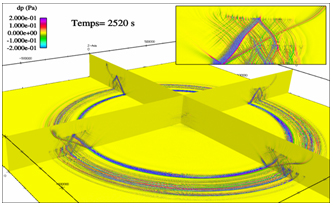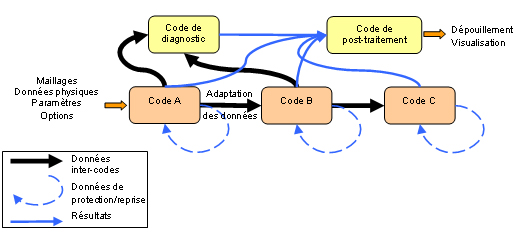Home / Software for HPC

## Software for HPC

It is often necessary to run a "numerical experiment"; a term which highlights the analogy between running a numerical simulation and performing a physical experiment. In a numerical simulation, the experimental apparatus consists of a collection of computer programs executed on computers.Simulation of the propagation of infrasound
2000 x 2000 x 200 km, 1010 elements

The computational software or "codes" are the translation, via numerical algorithms, of the mathematical equations of the physical models being investigated. Before and after the calculation, the environment software manages several complex operations for preparing the computations and their analysis.

Numerical simulation of complex multiple physical phenomena, whilst respecting the relevant scales in space and time, requires a large number of calculations to be performed which use and generate large volumes of data on powerful computers: this is high-performance computing.

### Modelling and applied mathematics

Numerical simulation involves using a computer to reproduce the functioning of a system, once it has been described by a set of models. It relies on specific mathematical and computational methods. At any point in the object being studied, several physical values (velocity, temperature, etc.) describe the state and evolution of the system. These are not independent. Rather they are linked and governed by equations which generally include partial derivatives. These equations represent the mathematical translation of the physical laws used to model the object's behaviour. Simulating the state of this object involves determining the numerical values of its parameters, ideally at all points. Since there are an infinite number of points, and hence an infinite number of values to calculate, this goal can only be achieved in special cases. A natural approximation is to only consider a finite number of points. There will then be only a finite number of values to calculate for the parameters and the required operations become accessible using a computer. The effective number of points considered, depends on the power of this computer: the greater the power, the better will be the final description of the object.

Underlying the calculation of these parameters and underlying numerical simulation therefore is the concept of reducing the infinite to the finite. This is known as discretisation.

### Codes

Codes lie at the heart of numerical simulation: they are the point where the various different and complementary specialisations come together. The physicist selects the models to study, the mathematician translates the model into equations and develops the algorithms to solve these equations, the computer specialist enables all of these algorithms to be executed on the computers and the engineer uses the codes to successfully carry out his project. A code is a complex assembly which, starting with the input parameters representing phenomena studied, delivers the results as output.
To anticipate and minimise the impact of computer upgrades, the codes are based on program architectures which enable each function to be more or less independent of the others.

A code represents a unit which capitalises on a wealth of knowledge built up over a long period of time. Its development and operation are managed as a project. A life cycle needs to be defined for it, starting from the schedule of conditions and continuing up to the delivery and maintenance of the deliverables and including specification, design, configuration management, coding, verification and validation, and documentation phases. This approach is a sign of quality for the end-users.

### Analysis and visualisation of results

Techniques and resources for exploitation of results from HPC calculations are one of the essential links in the numerical simulation chain. The final goal of these calculations is to understand the interactions of multiple physical phenomena. This understanding requires control of the complex and very large flows of data through a sequence of processing which ends with a graphic, interactive visualisation of the results.Computing sequence and data flows

Analysis of the results by exploitation of numerical databases comprises of several steps: selective extraction of the data (according to the physical parameter being investigated) and visualisation, extraction and transfer of the data for calculation and visualisation of the diagnostics.